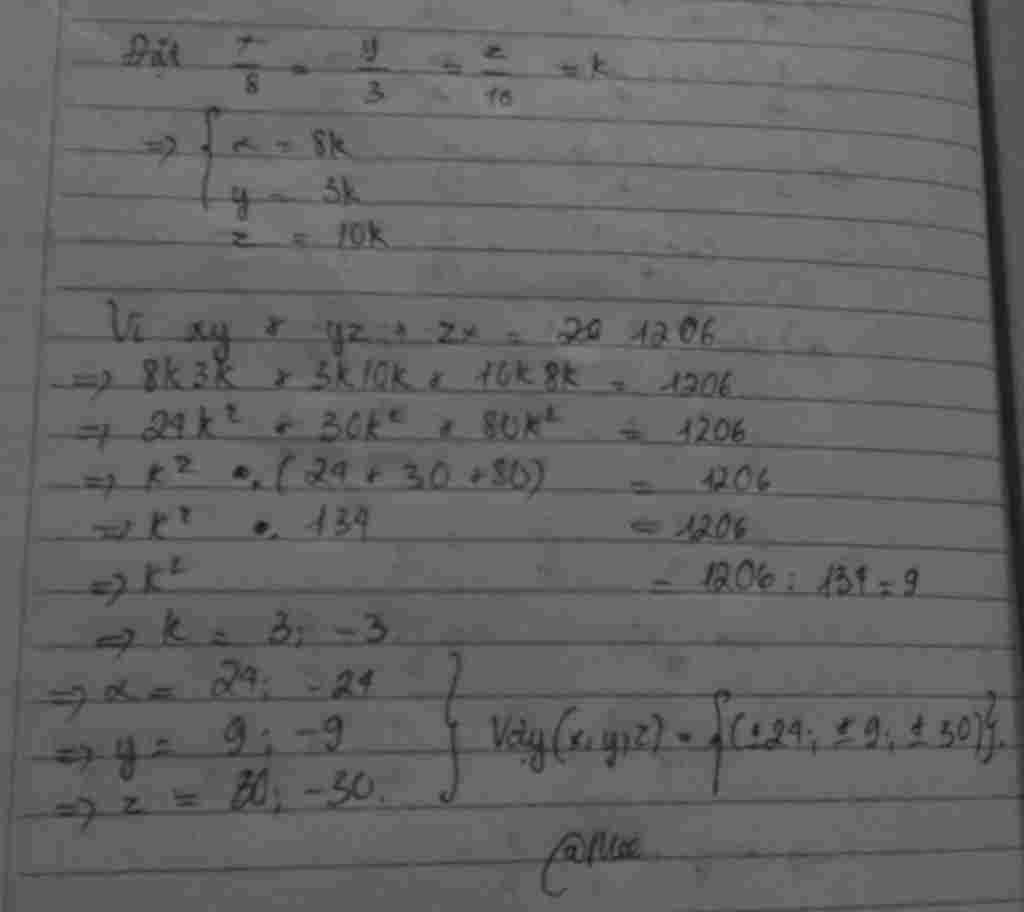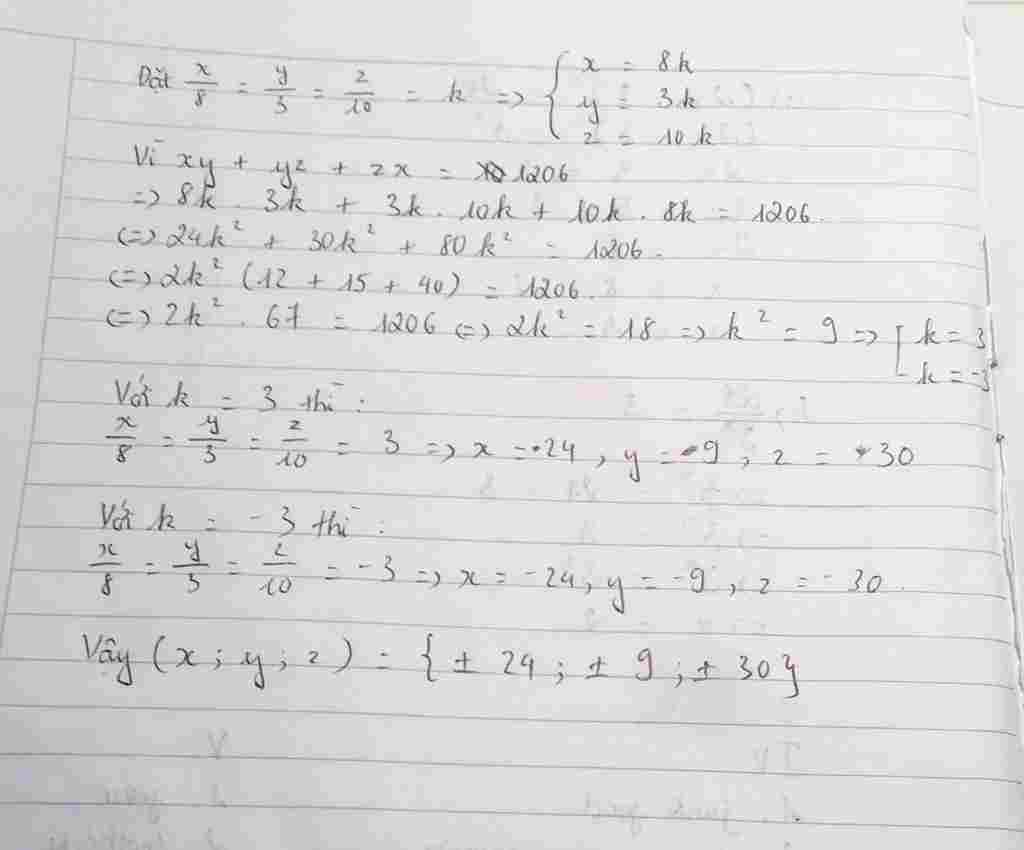Tìm x ; y ; z biết : $\frac{x}{8}$ = $\frac{y}{3}$ = $\frac{z}{10}$ và xy + yz + xz = 1206

Question

Tìm x ; y ; z biết :
$\frac{x}{8}$ = $\frac{y}{3}$ = $\frac{z}{10}$ và xy + yz + xz = 1206

in progress 0
1 year 2020-10-22T13:08:50+00:00 2 Answers 86 views 0